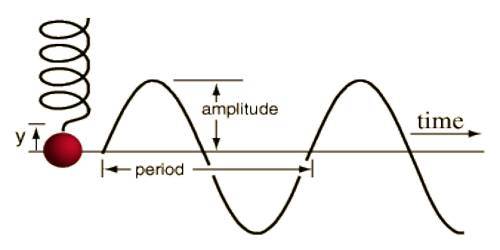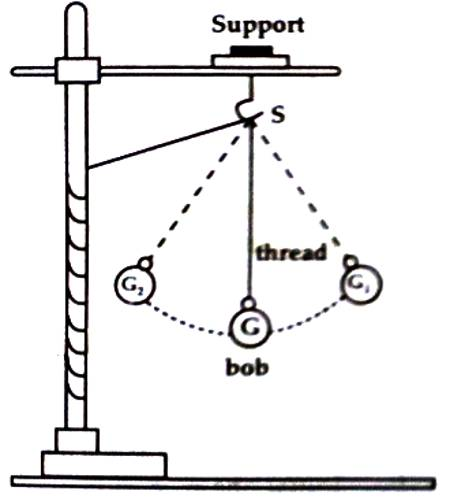# Simple Harmonic Motion

Simple Harmonic Motion

Discussion of simple harmonic motion is in important topic in physics. It is a special type of periodic motion. It is the simple form of harmonic motion. If has extensive use. Any complex oscillation can be expressed as the combination of many simple harmonic motion.

You have seen simple pendulum; when it oscillates, it oscillates to and fro [Figure]. If carefully observed you will see that when pulling the bob at one end and then released, you will see that it goes to the opposite direction i.e., it goes to the initial position. This type of motion is called simple harmonic motion.Definition: Simple harmonic motion is any motion where a restoring force is applied that is proportional to the displacement and in the opposite direction of that displacement.

It acceleration of a body executing periodic motion acts along a fixed point in its path of motion in such a way that its magnitude from that point is proportional to its displacement, then that motion of the body is called simple harmonic motion.